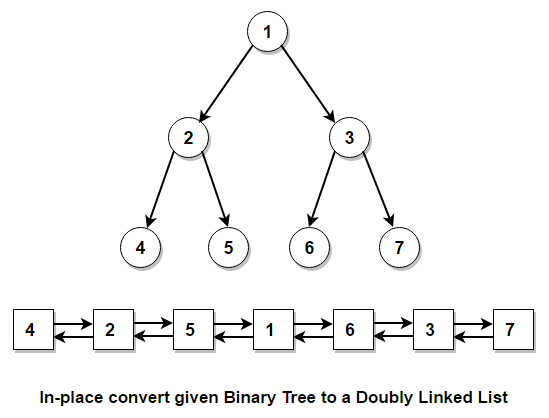# In-place convert convert given Binary Tree to Doubly Linked List

Given a binary tree, in-place convert it to a Doubly Linked List.

The left and right pointers of binary tree nodes should act as previous and next pointers in doubly linked list and the doubly linked list nodes should follow the same order of nodes as in-order traversal of the tree.

For example,Approach 1: (using in-order traversal)

The idea is to do a in-order traversal of the tree and for every node encountered, we insert it in the beginning of DLL. Since we are inserting the nodes in beginning of the doubly linked list, we need to reverse the linked list so that it follows the same order of nodes as in-order traversal of the tree.

C++ implementation –

The time complexity of above solution is O(n) and need O(h) extra space for the call stack where h is the height of the tree.

Approach 2: (using reverse in-order traversal)

Above approach requires two passes – one pass for converting binary tree to DLL and one pass to reverse the DDL. We can solve this problem in one traversal of the tree by doing reverse in-order traversal instead of normal in-order traversal. In reverse in-order traversal, we process the right subtree before left subtree. Now, the nodes will follow the order of in-order traversal.

C++ implementation –

The time complexity of above solution is O(n) and need O(h) extra space for the call stack where h is the height of the tree.

Approach 3: (keeping track of previous processed node in in-order traversal)

We can solve this problem in single traversal by doing in-order traversal only. The idea is to keep track of previous processed node in in-order traversal and for every encountered node, we set its left child to prev and prev’s right child to current node.

C++ implementation –

The time complexity of above solution is O(n) and need O(h) extra space for the call stack where h is the height of the tree.(1 votes, average: 5.00 out of 5)Loading...

Please use our online compiler to post code in comments. To contribute, get in touch with us.
Like us? Please spread the word and help us grow. Happy coding 🙂Subscribe
Notify ofGuest
AllergicToBitches

+1Guest

Thanks!Guest
ROSS MORRISON

JAVAAAAAAAAAAAAAAAAAAAAAAAAAAAAAAAAAAAAAAAAAAA